Journal DetailsFormat
Journal
First Published
09 Nov 2012
Publication timeframe
4 times per year
Languages
EnglishOpen Access

# Application of the thermoporoelasticity model in numerical modelling of underground coal gasification influence on the surrounding medium

###### Accepted: 14 Feb 2021
Journal DetailsFormat
Journal
First Published
09 Nov 2012
Publication timeframe
4 times per year
Languages
English

The purpose of this paper was to present the thermoporoelasticity model adapted for application in modelling processes, where phase transition may occur, such as during underground coal gasification (UCG). The mathematical model of the medium (soil/rock with pores filled with liquid/gas) in non-isothermal conditions is based on Biot's poroelasticity model. The poroelasticity model is expanded here by the influence of temperature and adjusted to the case where both liquid and highly compressible fluid are present in pores by using the gas laws. This requires considering temperature-dependent physical quantities such as pore fluid density, heat transfer coefficient and viscosity as functions of temperature. Based on the proposed mathematical model and the finite element method, a numerical model was built for the purpose of computing processes occurring in the vicinity of the UCG generator. The result of the authors’ work is a three-dimensional (3D) model, which was not only modified, but derived straight from the laws of thermodynamics, where fields of displacement, temperature and fluid flow are coupled. The model makes it possible to determine results significant to modelling of the UCG process, the reach of the gaseous phase's presence in pores, subsidence values, temperature distribution and directions and rate of seepage, without losing the simplicity and elegance of Biot's original concept. Next, the results of simulations for a hypothetical deposit to estimate the environmental impact of UCG are presented. After applying specific geometry and parameters, the model can be useful for verifying if the chosen technology of UCG in specific conditions will be safe for the environment and infrastructure.

#### Keywords

Introduction

The process of underground coal gasification, or UCG, is a technology for obtaining synthetic gas from deposits of hard coal or lignite in situ. UCG makes it possible to exploit deposits that would be unprofitable to extract by traditional methods: for example, deep or steep deposits with high ash content. It can also potentially be applied in the case of residual coal deposits in mines that have already been exploited [1, 2]; trials of such recovery are being conducted in mines in China . In Poland, within the framework of the HUGE and HUGE2 (Hydrogen Oriented UCG for Europe) programmes, UCG tests were undertaken in the mine “Barbara” ; successful experiments were also carried out by the Central Mining Institute and Academy of Mining and Metallurgy in the mine “Wieczorek” .

There are several UCG technologies; in general, they can be divided into the shaft (requiring human work underground) and shaftless (not requiring human work underground) methods. In shaftless methods (linked vertical wells, CRIP), there are usually drilled 2 boreholes (injection and production well), between which the UCG process is carried out. In modern methods, such CRIP (controlled retractable injection point) or P-CRIP (parallel CRIP), directional drilling is involved, and the point where the oxidant (air or oxygen with steam) is injected moves with the progress of the process, thus ensuring stable syngas quality. During UCG, processes take place that are analogous to those during traditional coal gasification over ground. Chemical reactions occur in the coal seam between coal and gases, and between the gases. Finally, the synthesis gas consists mainly of hydrogen, carbon oxides, methane and nitrogen. The proportions of these constituents will vary according to the seam's properties, the gasifying agent (oxidant), the amount of water used in the process and technology. A schematic of the UCG process is shown in figure 1.

In comparison to traditional deposit extraction methods, it is characterised by greater safety for the people employed during operation as well as less dust and noise pollution. It also makes it possible to reduce operating costs, including those generated by the transport and storage of coal, and does not require the storage of waste and ash . However, it poses a risk of polluting groundwater and air . Geotechnical issues involving analysis of the influence of the gasification process on the surrounding environment are an important part of the decision to undertake a project of this type, or whether to abandon activities in this respect. The influence of the installation on the environment must be carefully analysed in order to demonstrate that the given installation has no adverse impact on the natural environment .

In the gasification process, high temperatures are generated in the coal layer as a result of exothermic reactions, and this layer interacts strongly with the surrounding geological environment. The most important aspects from the perspective of environmental impact are subsidence, directions and rates of seepage and temperature distribution in the rock mass. The model used for simulations must account for coupled mechanical and thermal interactions as well as the possibility of phase changes in the fluid filling the medium's pores [10,11,12,13,14]. This article presents a coupled thermo-hydro-mechanical (THM) model based on Biot's poroelasticity model [15, 16].

The 3D consolidation theory, presented in isothermal form by Biot  and known as the poroelasticity theory, uses constitutive equations that make the strain of the medium and fluid discharge-dependent on stresses in both phases. It is applicable not only to soils or rocks, but also to porous media in general, including anisotropic media  such as concrete  or certain biological tissues [20, 21]. In the poroelasticity theory, the skeleton is treated as an elastic medium in which stress–strain relationships can be described by means of Hooke's law, and the pore pressure also has an influence on the skeleton's strain. The classical poroelasticity theory has been modified multiple times for the purpose of accounting for additional phenomena. Coussy [22, 23] uses changes in porosity in constitutive relationships instead of changes in fluid content. In addition, he distinguishes porosity in Lagrangian and Eulerian descriptions. Another form of the model is presented by Derski  and Strzelecki , who use the change in fluid volume instead of fluid content in the pores of the medium.

Using the poroelasticity model as a basis, there are also coupled THM models being built in various forms, depending on the phenomena analysed by the authors of these models. In Coussy's model , the state parameter used for creation of the model is porosity, but the final constitutive equations of the medium are transformed for using fluid content (depending on the volumetric strain of the skeleton, fluid pressure and temperature), so the model's structure is quite complicated. The model of Gawin et al.  focuses more on the flow and heat transfer of two immiscible fluids than on deformations. Coupling between the solid and fluid phase is implemented there by the Biot constant in the constitutive equation of the medium, which constant takes volumetric strain into account in both phases of the medium. In the heat conduction equations, stress is put on the effects related to the presence of 2 immiscible fluids; deformations of constituents are not taken into account there. The model of Bartlewska–Urban and Strzelecki  takes the rheological properties of the skeleton into account; it does not consider the presence of a gaseous phase in the pores of the medium. There is also a thermal seepage model  based on Biot's poroelasticity model, which takes volumetric strain into account. Liu et al.  presented the THM model with coupling implemented by the Biot constant for shale gas recovery, proving that gas recovery is strongly dependent on temperature.

In the case of elastic media, dependencies between temperature, strain and stress are described by Duhamel–Neumann relations. The method for deriving these relations was presented by Nowacki . The corresponding relations for poroelastic media can be derived analogously. In combination with the equation of fluid motion, they make up the thermoporoelasticity model. Numerical models applying different variants of the thermoporoelasticity model have been published, for example, in articles concerning geothermal springs , steam flooding of heavy oil reservoirs , the safety of storing radioactive waste , the influence of temperature changes on engineering structures , cement hydration  and ceramic filters . In this article, a proposal for a method of use for the thermoporoelasticity model, based on Derski's approach, for modelling coupled phenomena occurring in the area of coal gasification will be presented, using the example of a hypothetical brown coal deposit. The presented model concerns changes occurring in the vicinity of the reactor; process parameters such as gasification rate, temperature and pressure in the reactor are not modelled. Here, they constitute boundary conditions. A review of models used to simulate other processes occurring during UCG and their classification can be found elsewhere, for example, in the work of Rosen et al. .

Mathematical model
Equation of continuity

Equations of flow continuity in the thermoporoelasticity model are the same as in the classical poroelasticity model. The medium's equation of continuity is in the form : $DsρDt+ρε˙=−(ρlνir),i,$ {{{D^s}\rho } \over {Dt}} + \rho \dot \varepsilon = - \left( {{\rho _l}\nu _i^r} \right){,_i}, where ρ is the medium's density; ɛ is the skeleton's dilatation; ρl is the fluid's density; $νir$ \nu _i^r are components of the fluid's relative velocity of flow relative to the skeleton over the medium's surface; $DsDt$ {{{D^s}} \over {Dt}} is the material derivative; $DsDt=∂∂t+νr.is∂∂xi$ {{{D^s}} \over {Dt}} = {\partial \over {\partial t}} + \nu _{{\rm{r}}.i}^s{\partial \over {\partial {x^i}}} , where $νr.is$ \nu _{{\rm{r}}.i}^s are components of the skeleton's actual velocity. The fluid's equation of continuity, when we consider solely its motion relative to the skeleton, has the form: $Drρ2Dt+ρ2(θ˙−ε˙)=−(ρ2νr.is),i.$ {{{D^r}{\rho _2}} \over {Dt}} + {\rho _2}\left( {\dot \theta - \dot \varepsilon } \right) = - \left( {{\rho _2}\nu _{r.i}^s} \right){,_i}. where θ is the fluid's dilatation; ρ2 is the fluid's partial density; ρ2 = l where f is porosity; $νr.is$ \nu _{r.i}^s are components of the skeleton's actual velocity; $DrDt$ {{{D^r}} \over {Dt}} is the material derivative $(DrDt=∂∂t+νr.ir∂∂xi)$ \left( {{{{D^r}} \over {Dt}} - {\partial \over {\partial t}} + \nu _{r.i}^r{\partial \over {\partial {x^i}}}} \right) , where $νr.ir$ \nu _{r.i}^r are components of the fluid's actual velocity relative to the skeleton.

Conservation of momentum law

In the case of poroelastic media, mechanical coupling of phases in the conservation of momentum law is, for each of them, included in the form of kinetic energy and energy dissipation as a result of the fluid's flow through pores. The conservation of momentum law for the medium's solid phase is in the form: $∫SσijnjdS+∫ΩX(ρ−ρ2)dΩ=−∫ΩbνrdΩ+∫Ω(ρ11∂νrs∂t+ρ12∂νrf∂t)dΩ,$ \int\limits_S {{\sigma _{ij}}{n_j}dS + } \int\limits_\Omega {X\left( {\rho - {\rho _2}} \right)d\Omega = - \int\limits_\Omega {b{\nu ^{\rm{r}}}d\Omega } + \int\limits_\Omega {\left( {{\rho ^{11}}{{\partial \nu _r^s} \over {\partial t}} + {\rho ^{12}}{{\partial \nu _r^f} \over {\partial t}}} \right)} d\Omega ,} where $∫SσijnjdS$ \int\limits_S {{\sigma _{ij}}{n_j}dS} are surface forces acting on the skeleton, $∫ΩX(ρ−ρ2)dΩ$ \int\limits_\Omega {X\left( {\rho - {\rho _2}} \right)d\Omega } is the sum of body forces (X is acceleration), $−∫ΩbνrdΩ$ - \int\limits_\Omega {b{\nu ^r}d\Omega } are forces of viscous resistance (b is a coefficient of viscous resistance) and $∫Ω(ρ11∂νrs∂t+ρ12∂νrf∂t)dΩ$ \\int\limits_\Omega {\left( {{\rho ^{11}}{{\partial \nu _r^s} \over {\partial t}} + {\rho ^{12}}{{\partial \nu _r^f} \over {\partial t}}} \right)d\Omega } is inertia (ρ11 and ρ12 are mass coefficients, which take into account the fact that the relative fluid flow through the pores is not uniform ). For the fluid phase of the medium, the conservation of momentum law will take the form: $∫SσnidS+∫ΩXρ2dΩ=∫ΩbνrdΩ+∫Ω(ρ12∂νs∂t+ρ22∂νf∂t)dΩ$ \int\limits_S {\sigma {n_i}dS + } \int\limits_\Omega {X{\rho _2}d\Omega = } \int\limits_\Omega {b{\nu ^r}d\Omega + } \int\limits_\Omega {\left( {{\rho ^{12}}{{\partial {\nu ^s}} \over {\partial t}} + {\rho ^{22}}{{\partial {\nu ^f}} \over {\partial t}}} \right)d\Omega } where σ is partial stress in the fluid (σ = −pf). After applying the Gauss–Ostrogradsky theorem, in the quasi-static case (accelerations of both phases are small, hence $∂ν∂t=0$ {{\partial \nu } \over {\partial t}} = 0 ), equations (3) and (4) take the form: $σij,j+σ,i+Xρ=0,σ,i+Xρ2=bνr.$ \matrix{ {{\sigma _{ij,j}} + {\sigma _{,i}} + X\rho = 0,} \hfill \cr {{\sigma _{,i}} + X{\rho _2} = b{\nu ^r}.} \hfill \cr } The fluid's relative velocity can be obtained from the second of the equations: $νir=−f2ρlgbH,i$ \nu _i^r = - {{{f^2}{\rho _l}g} \over b}{H_{,i}} Corresponding to Darcy's law, $νir=−kH,i$ \nu _i^r = - k{H_{,i}} , where k is conductivity and H is hydraulic head. It is assumed that the coal bed is not fractured; otherwise, Forchheimer's formula should be used with permeability as a function of stress .

Constitutive equations

Non-isothermal models of the biphasic medium are divided into two categories: LTE (local thermal equilibrium) – where the influence of the medium's mean temperature is accounted for in constitutive relations, and LTNE (local thermal non-equilibrium), where we consider the influence of the skeleton's and fluid's temperatures separately. In the event of an identical temperature for both phases, the results of both models are the same. The authors created both LTE and LTNE models, which were described and discussed in more detail in the works [15, 41, 42]. In this article, due to the scale of the phenomenon, the numerical model was based on the LTE approach. The procedure for deriving constitutive equations and heat transfer equations for the model are presented below in shortened form. Constitutive relations for the poroelastic medium under non-isothermal conditions can be obtained by accepting Helmholtz free energy χ as the state function, and the medium's strain, fluid's strain and temperature χ =χ (ɛij, θ, T) as state parameters . According to the first law of thermodynamics, a change in the medium's internal energy will be equal to $w˙=σijε˙ij+σθ˙−qi,i$ \dot w = {\sigma _{ij}}{\dot \varepsilon _{ij}} + \sigma \dot \theta - {q_{i,i}} . Using the definition of free energy and entropy, the following dependencies can be obtained: $σij=∂χ∂εij, σ=∂χ∂θ, −s=∂χ∂T.$ {\sigma _{ij}} = {{\partial \chi } \over {\partial {\varepsilon _{ij}}}},\,\sigma = {{\partial \chi } \over {\partial \theta }},\, - s = {{\partial \chi } \over {\partial T}}.\,\, By differentiating the free energy's Taylor series expansion with accuracy to small values of the second order, relations can be obtained in the form corresponding to the one proposed by Strzelecki , additionally expanded by the influence of temperature: $σij=2Nεij+(Aε+Qθ−(3Kαts+QαTl)ϑ)δij,σ=Qε+Rθ−(3QαTs+RαTl)ϑ,−s=−(3Kαts+QαTl)ε−(3QαTs+RαTl)θ+ξϑ.$ \matrix{ {{\sigma _{ij}} = 2N{\varepsilon _{ij}} + \left( {A\varepsilon + Q\theta - \left( {3K\alpha _t^s + Q\alpha _T^l} \right)\vartheta } \right){\delta _{ij}},} \cr {\sigma = Q\varepsilon + R\theta - \left( {3Q\alpha _T^s + R\alpha _T^l} \right)\vartheta ,} \cr { - s = - \left( {3K\alpha _t^s + Q\alpha _T^l} \right)\varepsilon - \left( {3Q\alpha _T^s + R\alpha _T^l} \right)\theta + \xi \vartheta .} \cr } where $αts$ \alpha _t^s and $αTl$ \alpha _T^l are, respectively, the skeleton's (linear) and fluid's (volumetric) thermal expansion coefficients, ξ is a coefficient describing the second derivative of free energy with respect to temperature, N and A are the Lamé parameters μ and λ for the skeleton of the porous medium, Q is the coupling coefficient resulting from the interaction between the solid and liquid phases. The fluid's compressibility is accounted for in this model as R−1. While water is a fluid of low compressibility, gas is a highly compressible fluid; moreover, its volume is strongly dependent on temperature. Relationships between pressure, volume and temperature are described by gas laws. By using the Boyle–Mariotte law pV const, partial stress in the fluid can be written as a function of strain: $σ=σa1+θ.$ \sigma = {{{\sigma _a}} \over {1 + \theta }}. where σa is partial stress in the fluid resulting from initial pressure. Hence, constitutive equations of the biphasic medium filled with gas take the form: $σij=2Nεij+(Aε+Qθ−(3Kαs+QT)ϑ)δij,σ=Qε+σa(1+θ)fθ−(3Qαs+σa(1+θ)fT)ϑ.$ \matrix{ {{\sigma _{ij}} = 2N{\varepsilon _{ij}} + \left( {A\varepsilon + Q\theta - \left( {3K{\alpha ^s} + {Q \over T}} \right)\vartheta } \right){\delta _{ij}},} \cr {\sigma = Q\varepsilon + {{{\sigma _a}} \over {\left( {1 + \theta } \right)f}}\theta - \left( {3Q{\alpha ^s} + {{{\sigma _a}} \over {\left( {1 + \theta } \right)fT}}} \right)\vartheta .} \cr }

Heat transfer equations

Heat transfer equations were obtained by using the entropy equation. The ξ coefficient can be determined under the assumption of constant strain of the fluid and skeleton, by using the definition of specific heat in the case of constant volume. Thus, the final heat transfer equation can be written in the form: $λ∇2T=−(3Kαts+QαTl)ε˙T−(3QαTs+RαTl)θ˙T−ρcνT0ϑ˙T.$ \lambda {\nabla ^2}T = - \left( {3K\alpha _t^s + Q\alpha _T^l} \right)\dot \varepsilon T - \left( {3Q\alpha _T^s + R\alpha _T^l} \right)\dot \theta T - {{\rho {c_\nu }} \over {{T_0}}}\dot \vartheta T. In the case of the gas in the medium's pores, the equation will take the form: $λ∇2T=−(3Kαs+QT)ε˙T−(3Qαs+σa(1+θ)fT)θ˙T−ρcνT0ϑ˙T,$ \lambda {\nabla ^2}T = - \left( {3K{\alpha ^s} + {Q \over T}} \right)\dot \varepsilon T - \left( {3Q{\alpha ^s} + {{{\sigma _a}} \over {\left( {1 + \theta } \right)fT}}} \right)\dot \theta T - {{\rho {c_\nu }} \over {{T_0}}}\dot \vartheta T, where λ is heat transfer coefficient, and cv is isochoric specific heat. The mathematical model presented above describes a coupled THM field; owing to use volumetric strain of both phases and entropy as state parameters, it is characterised by its simple formulation – individual impacts are taken into account in subsequent terms of the constitutive equations. Owing to the use of a full Biot model, rather than a simplified version, the model enables analysis of shear strain, as well as taking into account the possible presence of highly compressible fluid. Despite its simple composition, the model is strongly non-linear due to the fact that many of parameters are not material constants, but functions of temperature and pressure, which is an obstacle in finding the analytical solution. Results of smaller-scale and complexity numerical models give results close to [28, 29], with differences resulting from phenomena, simplifications and material properties taken into account in models, which suggests that the model is correct.

Numerical model
Geometry of the model

As an example, we use one of deposits of brown coal, which is not being exploited due to geological conditions and environmental issues [45, 46]. It was assumed that part of the brown coal deposit serving as an example, with a thickness of 3 m, deposited at a depth of approximately 120 m below the ground level, would be gasified. Layers of clay below and above the layer of coal are insulating the deposit, and the model contains 8 geological layers, with boundaries whose ordinates are known at 16 points distributed over a 500-m mesh. The model's geometry is shown in figure 2.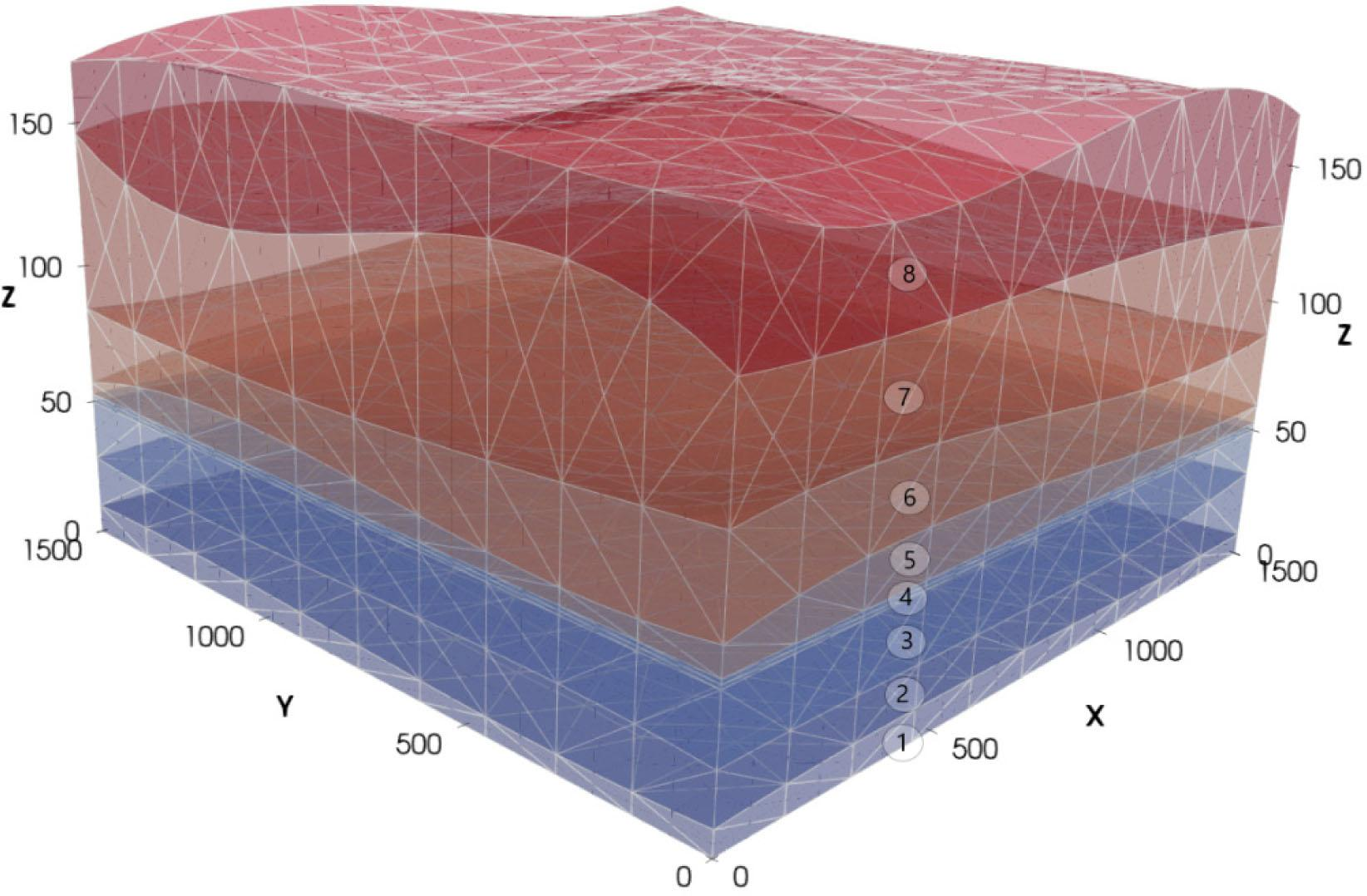Generated finite element mesh determining the model's geometry (non-uniform scale, aspect ratio 5:1). Geological layers: 1. sandstone, 2. clayey pebble, 3. clay, 4. lignite (UCG generator is located in the middle of this layer), 5. clay, 6. clayey sand, 7. clay, 8. silty clay.

A plane within the sandstones was set as the “0” ordinate, being the bottom of the model. It was assumed that the area in which gasification takes place is found in the central part of the model and has dimensions of 10 m * 100 m (figure 3).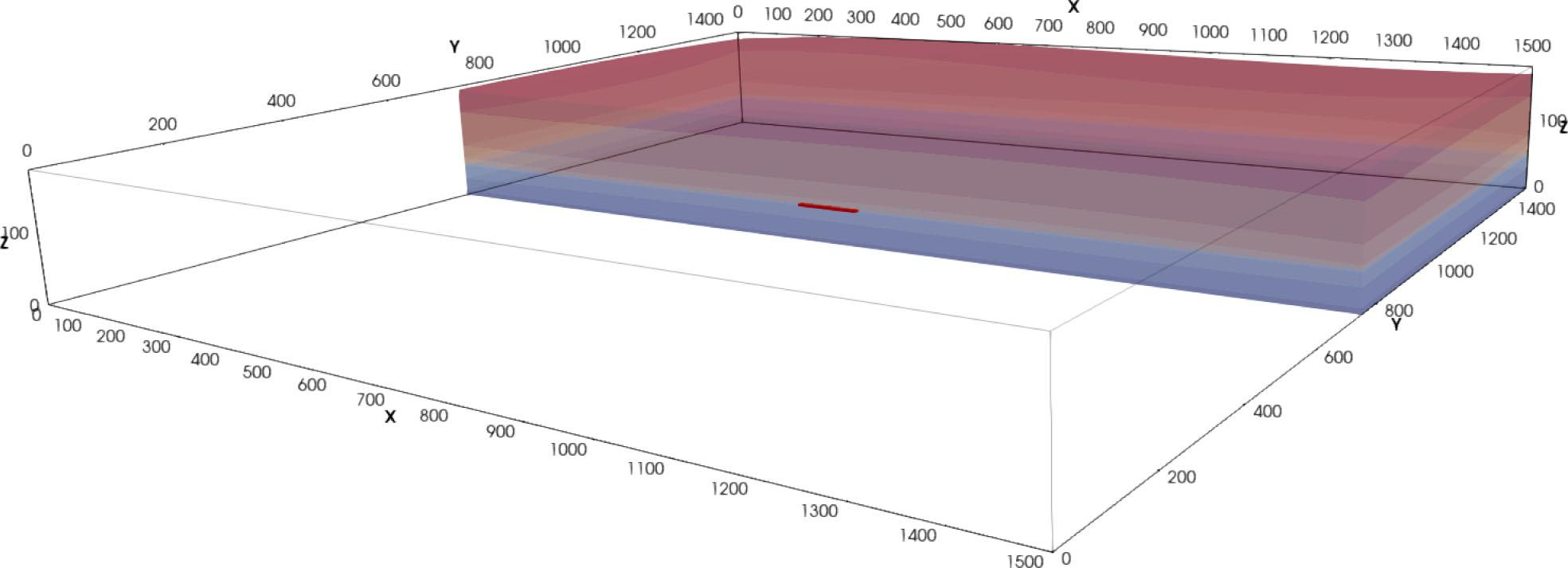Cross-section (y = 750 m) through the area of interest – position of coal gasification generator marked at the centre.
Parameters

Examples based on data from the literature concerning the deposit  and material tables containing the mechanical and thermal parameters of soils were adopted for simulations. After the end of the gasification process, it was assumed that the coal's mechanical parameters would decline. The parameters adopted for simulations are given in tables 1 and 2.

Material parameter values of individual geological layers.

Layer Roof min [m] Roof max [m] f [−] N [Pa] A [Pa] α [−] ρs [Mg/m3] K [m/s] cv [J/kg/K] λ [W/m/K]
Sandstone 4.7 10.9 0.15 2.31E+09 3.46E+09 1.16E-05 2.6 5.00E-07 1100 3.1
Clayey pebble 25.2 32.7 0.28 3.90E+06 2.40E+07 6.00E-06 2.53 3.00E-06 639 2.5
Clay 50 50 0.31 1.32E+07 6.91E+07 5.80E-06 2.38 3.00E-08 566 2.1
Coal* 53 53 0.2 5.80E+08 8.70E+08 5.00E-06 1.21 5.00E-09 1250 0.5
Clay 57.2 69.8 0.4 1.28E+06 9.42E+06 5.90E-06 2.62 6.00E-08 481 2.9
Clayey sand 80.5 95 0.32 1.30E+06 9.00E+06 6.00E-06 2.49 1.20E-06 889 3.5
Clay 125.9 149.9 0.37 2.50E+06 1.85E+07 6.10E-06 2.71 7.00E-08 514 1.4
Silty clay 166.1 184 0.28 3.30E+06 1.50E+07 6.00E-06 2.66 5.00E-08 612 1.9

initial values, which gradually fall to 0.1% of the initial value in the area of the gasification generator due to loss of mass of coal during the process

Thermal parameters of individual pore fluids and medium.

Thermal expansion coefficient 1) [1/K] Initial heat transfer coefficient [W/m/K] specific heat [J/kg/K]
Water 69·10−6 0.6 4150
Water vapour 1/T 16.2·10−3 1970
Medium - λ = (1 − f) λs + f cν = (ρ1 cνs + ρ2 cνf) / ρ

Volumetric expandability is given for fluids, linear for the skeleton.

Temperature-dependent value, initial value (for 0°C)

The medium's heat transfer coefficient and specific heat depend on the phase of the pore fluid and, in addition, the parameters of a given phase change with temperature. The permeability coefficient is dependent on viscosity: (k = K/μ), which changes with temperature. The dependency of water viscosity with respect to temperature is described by the Thorpe–Rodgers formula: $μw=1.79⋅10−31+3.37⋅10−2(T−273)+2.2⋅10−4(T−273)2.$ {\mu _w} = {{1.79 \cdot {{10}^{ - 3}}} \over {1 + 3.37 \cdot {{10}^{ - 2}}\left( {T - 273} \right) + 2.2 \cdot {{10}^{ - 4}}{{\left( {T - 273} \right)}^2}}}. In the case of gases, viscosity is described by Sutherland's formula: $μg=μ0273+CsT+Cs(T273)1.5,$ {\mu _g} = {\mu _0}{{273 + {C_s}} \over {T + C_s}}{\left( {{T \over {273}}} \right)^{1.5}}, where μ0 is initial viscosity, and Cs is Sutherland's constant, for water vapour μ0 = 8.53·10−6 Pa·s, Cs = 673 for air μ0 = 17.21·10−6 Pa·s Cs = 122. Thermal parameters for both pore fluids are given in table 2.

Similar to the case with viscosity, Sutherland's formula describes the dependency of gas thermal conductivity with respect to temperature: $λ=λ0273+CsT+Cs(T273)1.5,$ \lambda = {\lambda _0}{{273 + {C_s}} \over {T + {C_s}}}{\left( {{T \over {273}}} \right)^{1.5}}, where λ0 is the initial heat transfer coefficient. In the cases of both gases and water, water density was modelled as dependent on temperature and pressure. In the case of water, density is described by the formula: $ρw=ρ0[1+αw(T−Tp)](1−βwp),$ {\rho _w} = {{{\rho _0}} \over {\left[ {1 + {\alpha ^w}\left( {T - {T_p}} \right)} \right]\left( {1 - {\beta _w}p} \right)}}, where ρ0 = 1000kg / m3, while the density of gases is described by a function arising from the Clapeyron equation: $ρg=prT,$ {\rho _g} = {p \over {rT}}, where r is the individual gas constant for water vapour r = 461.5 J/kg/K.

The numerical model for FEM (finite element method) simulations was created using FlexPDE v.6 software. It was assumed that water is initially the pore fluid throughout the entire area, and as temperature grows, it undergoes phase transformation in certain areas. To determine the phase transformation temperature depending on the pressure in the medium, the Clausius–Clapeyron equation was used: $Tp=T0LL−T0Rln(pp0).$ {T_p} = {{{T_0}L} \over {L - {T_0}R \ln \left( {{p \over {p_0}}} \right)}}. where L is specific latent heat, T is temperature, p pressure p0 initial pressure, R is the gas constant. The mathematical model of thermo-consolidation for a porous solid–gas system was applied in the area where phase transformation occurred.

The use of different constitutive relations and parameters within a single model was made possible by the construction of a script using a coefficient defining the current state of matter, equal to 1 or 0, by which values pertaining to the given state of matter were multiplied (both values were computed), for example, in the case of fluid dilatation: θ = θgas (1 − β) + θliquidβ.

Model was run in 3 versions:

For the space, where gas is present in the pores, all mentioned modifications were applied: constitutive equations (10), conductivity and thermal parameters.

Constitutive equations without modification (8), change in conductivities applied.

No modifications in constitutive equations nor other parameters.

Our basic version is A. All the figures concern version A, unless version B or C is marked in the description. Versions B and C are used for comparison and identification of impact of given modifications.

Boundary conditions and initial condition

Strain: The absence of displacements in the model's bottom plane and the absence of horizontal displacements in side planes was assumed. The condition of conformity of horizontal and vertical displacements was accepted on individual dividing surfaces between layers. Initial displacements are equal to zero, and initial stress results only from the mass of overburden.

Pressure: The absence of fluid flow through the bottom and side surfaces of the model was assumed, and the condition of conformity of fluid pressure on surfaces dividing layers was adopted.

Temperature: The known temperature at ground level and on the side and bottom planes was accepted, changing linearly depending on depth: from 10° C at ground level to 15° C at a depth of 180 m below ground level (Fig. 4).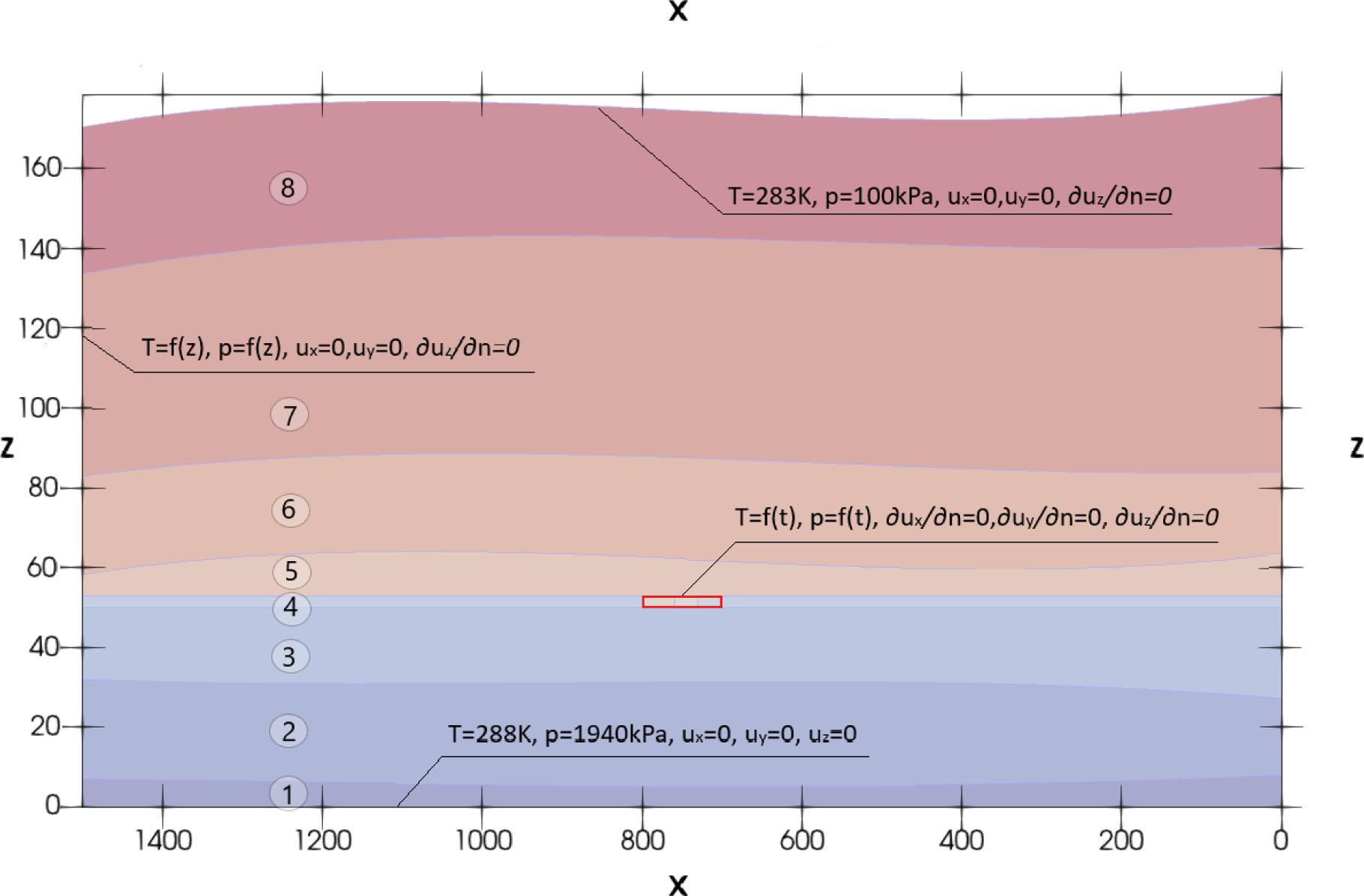Main boundary conditions on cross-section, (non-uniform scale, aspect ratio5:1). Geological layers: 1. sandstone, 2. clayey pebble, 3. clay, 4. lignite (UCG generator is located in this layer), 5. clay, 6. clayey sand, 7. clay, 8. silty clay.

A gasification process scenario based on descriptions of coal gasification in the literature [7, 47] was adopted for the coal gasification generator. 2 years was accepted as the duration of the coal gasification period. Temperature increases gradually, as the UCG generator is accepted as a heat source. Then, as the gasification process progresses along the coal layer, heat is being dissipated. The evolution of temperature in exemplary points (x = 715, 745 and 775m) belonging to the gasified area (x = 700 to 800m) is shown in fig. 5. It was assumed that pressure reduced with respect to hydrostatic pressure will be maintained in the reactor over the course of the gasification process – this is one of approaches to UCG, applied to ensure that water is present in the process and to prevent the escape of gas and pollution [9, 48].

Displacements and pressure distribution of pore fluid resulting from self-weight were adopted as the initial condition, and temperature distribution changes linearly from 10° C to 15° C, similar to the case with the boundary conditions. Simulations were carried out in two stages: initially, only self-weight load without temperature changes was assumed, then the obtained displacement results were taken as the initial condition for the second stage of simulations in order to observe solely the effects of gasification.

Results of numerical simulations

Conducted numerical simulations made it possible to obtain fields of the medium's displacement, of stresses in the fluid and of temperature. This made it possible to determine the directions and rate of seepage, thermal flux and stresses in the rock mass. Thanks to the knowledge of the distribution of temperature and pressure in the rock mass, it was possible to determine the reach of gas presence in the medium's pores. In addition, numerical simulations made it possible to analyse temperature- and/or pressure-dependent parameters such as fluid density, conductivity coefficient and heat transfer coefficient. The FlexPDE program was chosen for the calculations because it enables any differential equation to be solved, and the number of simultaneously solved equations is not limited; it enables vector and array variables and is able to control timestep and automatically refines mesh – it monitors accuracy, finds areas with high error values and refines their mesh (user can also force mesh refining with a function – here on the front defined by the Clausius–Clapeyron equation). During simulations, the number of nodes ranged from 42,752 to 88,390 and the number of elements from 29,815 to 63,185 due to the mesh refinement in the area of interest.

The temperature distribution in the surroundings of the gasified deposit at certain instances of time is given in fig. 6a–f. As can be seen, the temperature maximum moves along the gasified layer of coal over time. The rock mass cooling process is slow, and elevated temperature around the deposit persists for many years after the process is completed.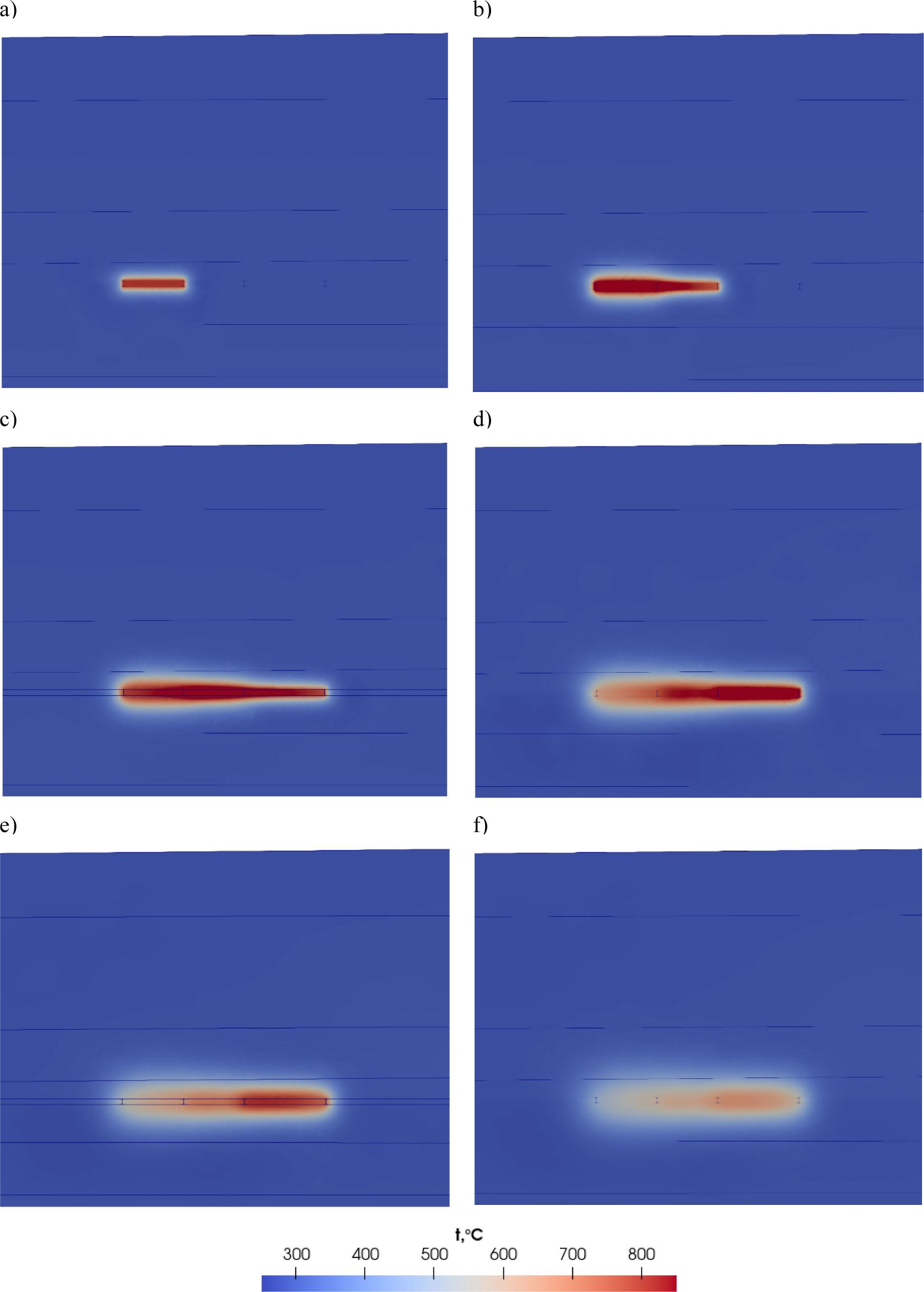Evolution of temperature changes around the coal gasification generator over time: a) start, b) 0.5 year, c) 1 year, d) 1.5 year, e) 2 year, f) 2.5 year.

Phase transition occurs as a result of the elevated temperature in the vicinity of the gasification area. The reach of phase transition is greatest at time 1.5 year – its reach is approximately 10 m outside the generator. It is possible to identify this region also in versions B and C, but in versions A and B, the temperature differences are small, and in version C, it is not necessary, because parameters do not change. Fig. 7a–f presents the area where water vapour is present at points in time corresponding to those given in fig. 6. A spatial view of the situation of the area in which gas (water vapour) is present in the medium's pores is shown in fig. 8 (example at t = 1 year).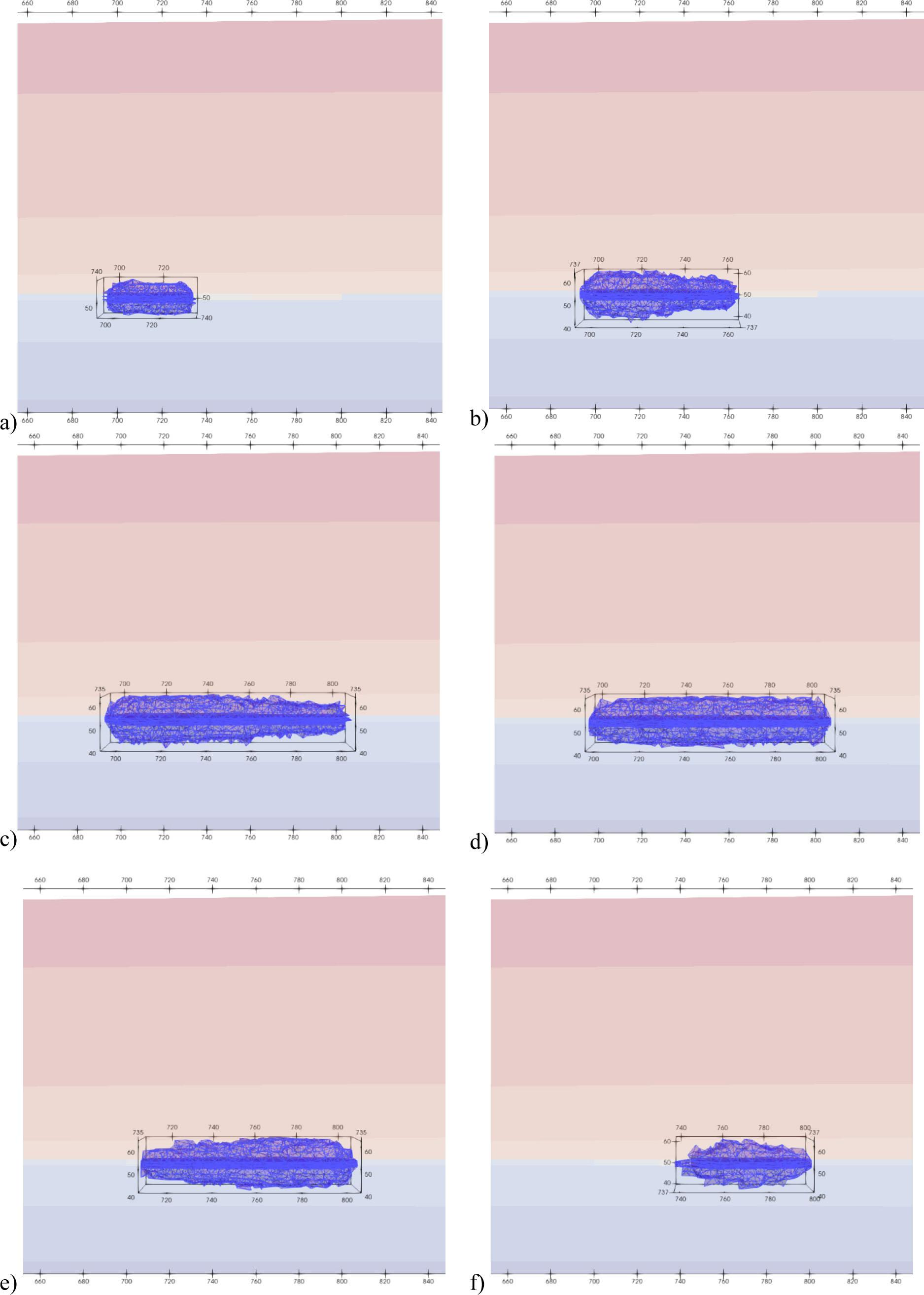Water vapour presence in cross-section at times a) start, b) 0.5 year, c) 1 year, d) 1.5 year, e) 2 years f) 2.5 year.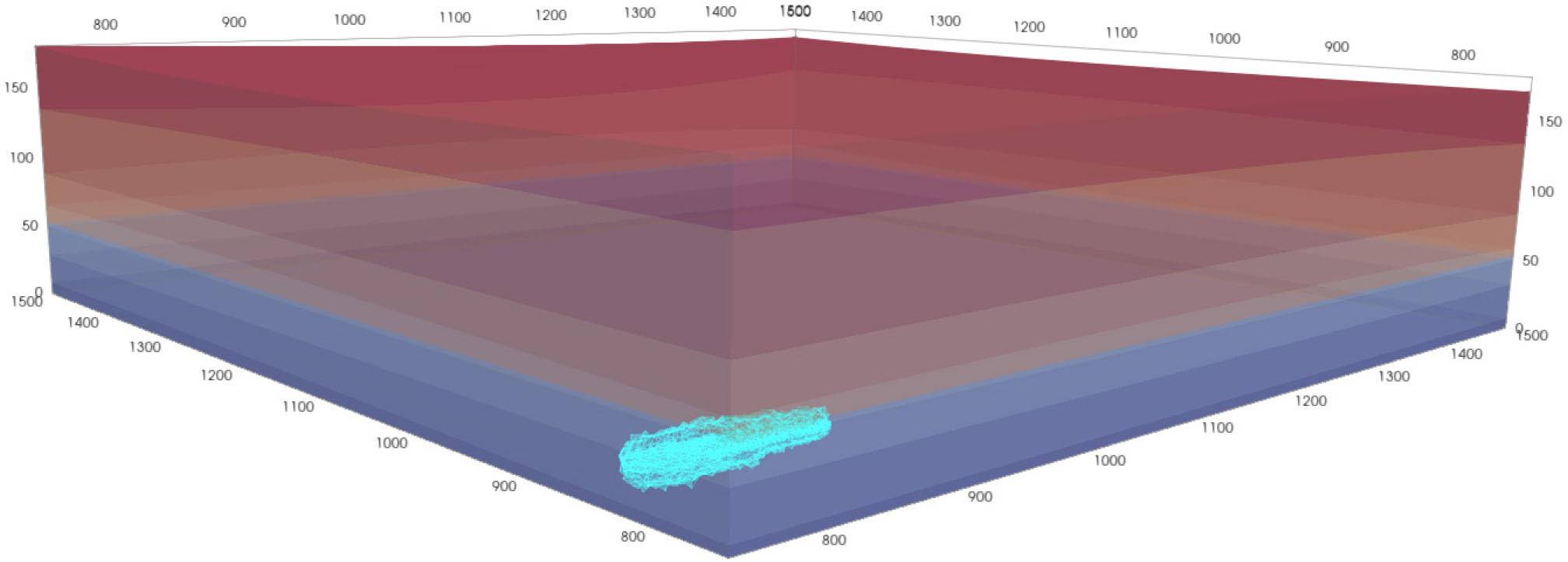Spatial situation of area of water vapour presence at time t = 1 year.

The vector field of displacements resulting from elevated temperature is shown in fig. 9a–d. These displacements are the result of elevated temperature and changes in the coal layer's mechanical parameters due to gasification (table 1). Maximum displacements caused by elevated temperature amount to +0.14 m and occur near the roof of coal at time t = 1 month from the start of the gasification process, then subsidence starts due to the reduction of coal mechanical parameters.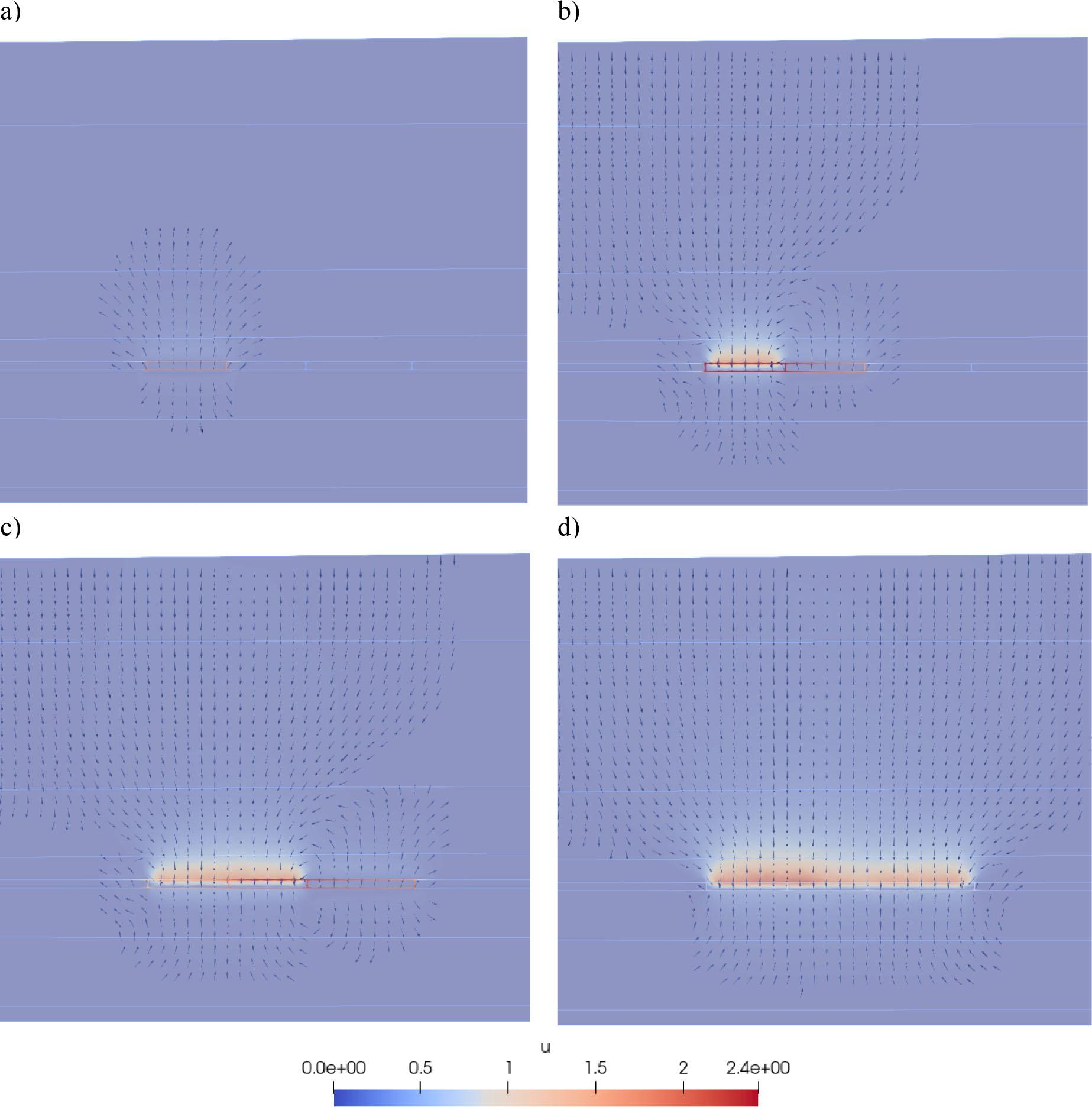Distribution of displacements (m) in the cross-section at y = 750 m at times a) 1 month, b) 0.5 year, c) 1 year, d)2 years.

Over time, small displacement values also appear at a greater distance from the gasified deposit, also at the ground level. Fig. 9a–d shows the vertical displacements in the cross-section over the course of the gasification process, initially positive as a result of thermal expansion, then negative due to subsidence after the end of the process, caused by the reduction of mechanical parameters. Final displacements of the ground level amounted to −5 cm in all versions (A, B and C). The cross-sections of displacements for model with modified constitutive equations (A) and constitutive equations of water (B) at selected instants above the deposit (y = 750, z = 58) is shown in fig 10a. The spatial distribution of displacements (A) on the background of the temperature field are shown in fig. 10b and 10c. Time instants t = 1 month, and the final instant of modelling, were selected. In figure 10c, besides displacements caused by thermal expansion, oppositely oriented displacements can be seen in the first section of the gasified deposit resulting from changes to the medium's mechanical parameters. On the cross-sections can be seen difference between behaviour of medium with modified constitutive equations (A – gas is present in the pores during the process of gasification) and with constitutive equations of water (B) – medium filled with gas deforms faster, but the final displacements are the same for both cases (−1.4 m). Subsidence evolves faster in the case A – 4 cm after 1.5 year (2 cm for version B).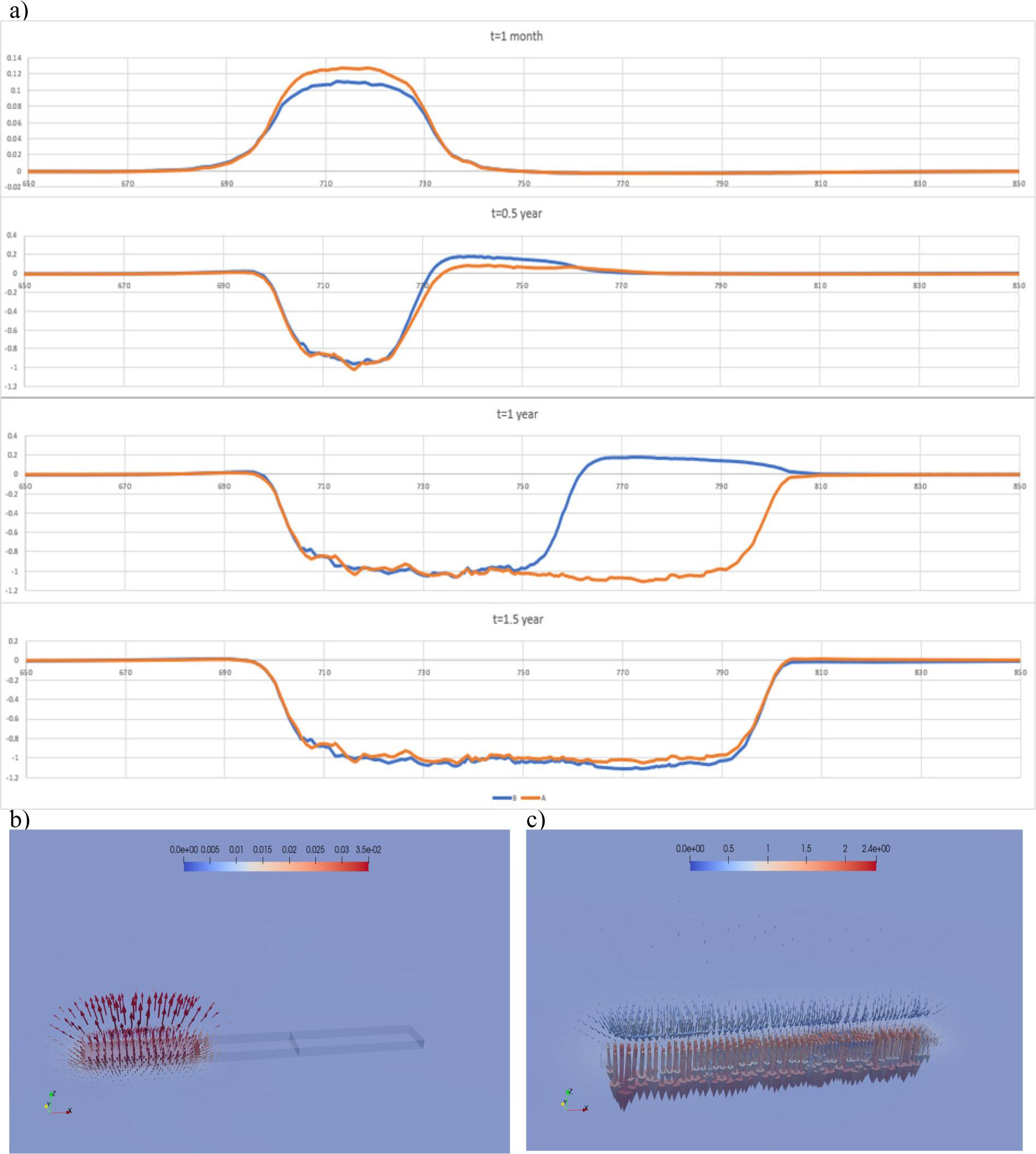a) Charts of displacements (m) over time, b) 3D vector field of displacements near the generator at t = start, c) displacement vector field near the generator at time t = 2 years.

Horizontal displacements are small compared to vertical displacements. Similar to the vertical displacements, the greatest values of displacements caused by temperature are reached in the layer of clays above the coal deposit. The distribution of horizontal displacement vectors in cross-section z = 51.5 at time t = 1.5 year (greatest displacements) is shown in fig. 11.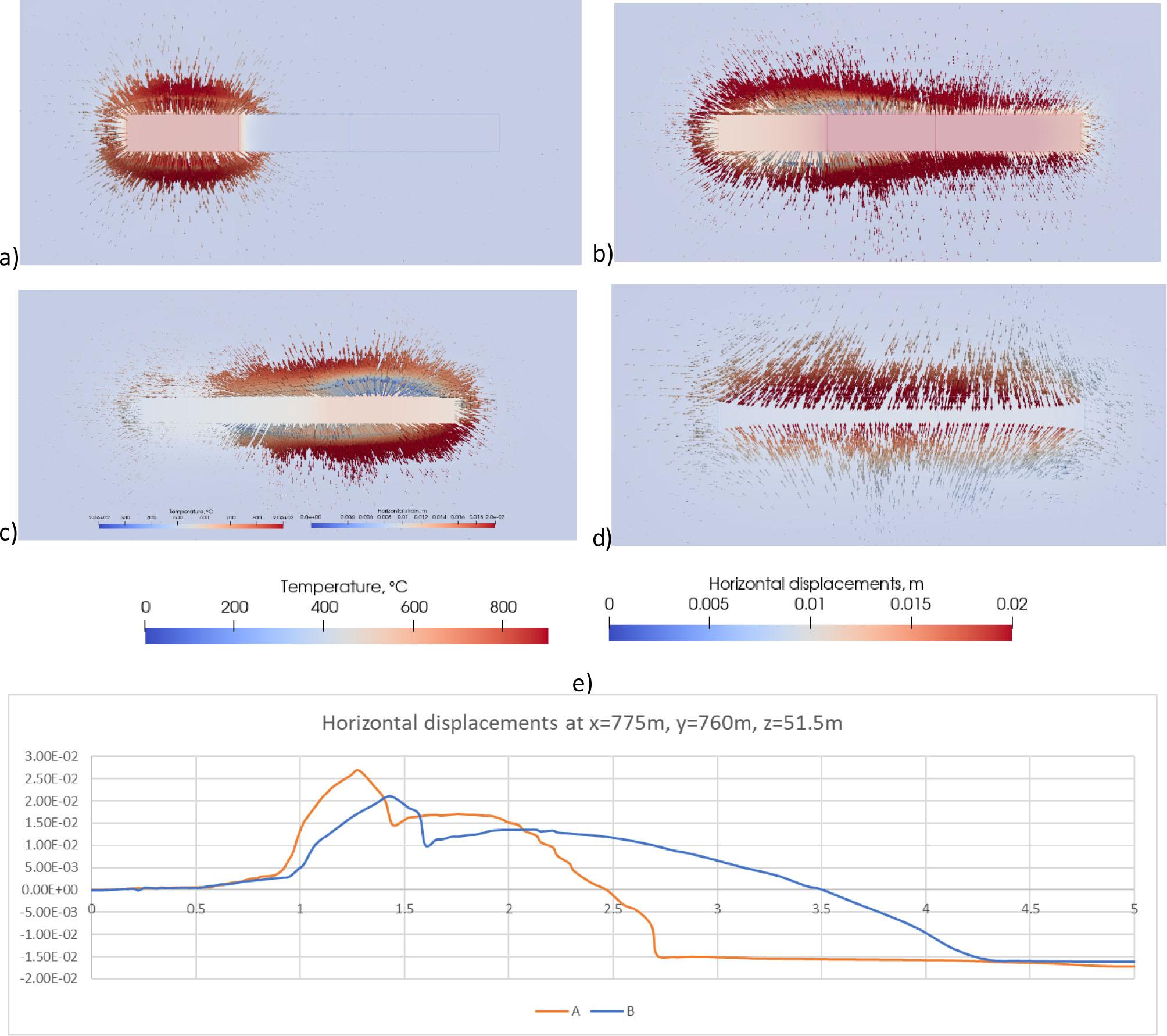Vector field of horizontal displacements (m): a–d) on the background of the temperature in cross-section z = 51.5: a) 1 month, b) 1 year c) 2 year, d) 3 year e) horizontal displacements over time (A and B).

In the gas-filled space, conductivity is lower and seepage rate is higher due to its low viscosity with respect to water. According to Sutherland's formula, the viscosity of gas increases as temperature rises, while conductivity decreases.

Water seepage rate vectors at t = 1.5 year are shown in fig. 13 – their direction indicates that water reaches the gasification area according to the assumptions of the reduced-pressure method of coal gasification, providing the water vapour required for the process and also preventing migration of pollution. In case of no change in parameters (version C), seepage rates are smaller in the volume, where phase transition occurred, but change of rates outside this region is negligible.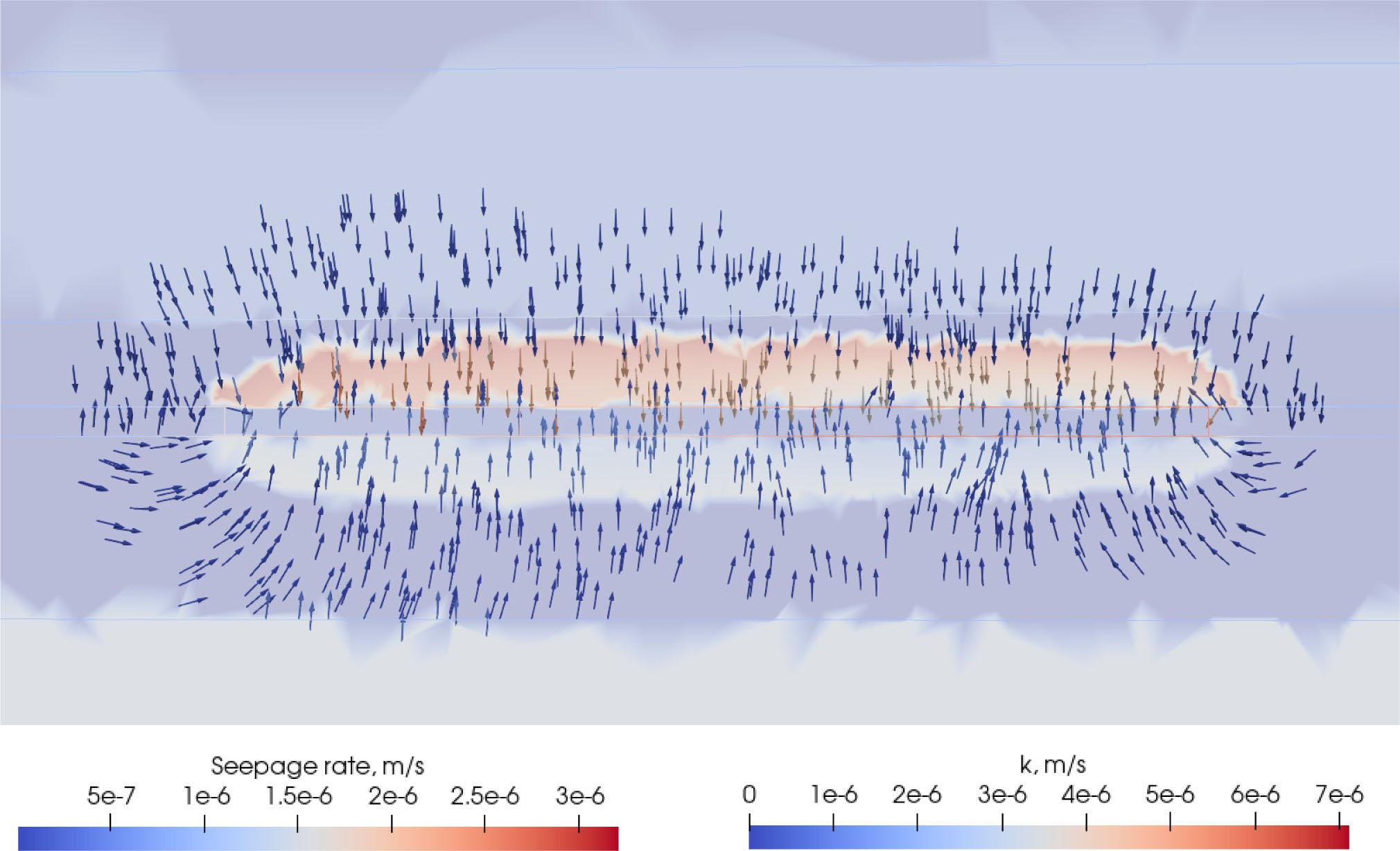Vector field of water conduction rate (m/s) on the background of the permeability (m/s) in cross-section y = 750 m at t = 1.5 year.

The distribution of heat transfer coefficients at time t = 1 year is presented in figure 14. It shows a difference between the hot, gas-filled area and the cooler, liquid-filled area, which is due to lower heat transfer through gas and change in thermal conductivity along with temperature (the cross-section was made accounting for the shapes of finite elements). The difference in thermal conductivity is visible only in the closest proximity to the coal gasification generator, and its influence is relatively small, which coincides with the conclusions of Otto and Kempka .

However, in unsteady conditions, one must take into account also the specific heat. After the heat source is turned off, in case of water vapour in the pores, temperature drops relatively fast, while liquid water stores heat for many years until it is dissipated in the rock mass. The amount of heat needed to maintain temperature of the process during the process is also smaller after phase transition. Figure 15 shows temperature field in 6th year of simulation and the course of temperature in point 71,575,063 (10 m above the generator) in variant A (water vapour in pores) an variant C (no distinction between parameters of gas and liquid). The maximum temperature in variant C is 10°C higher due to thermal inertia and lack of insulating layer with gas in pores.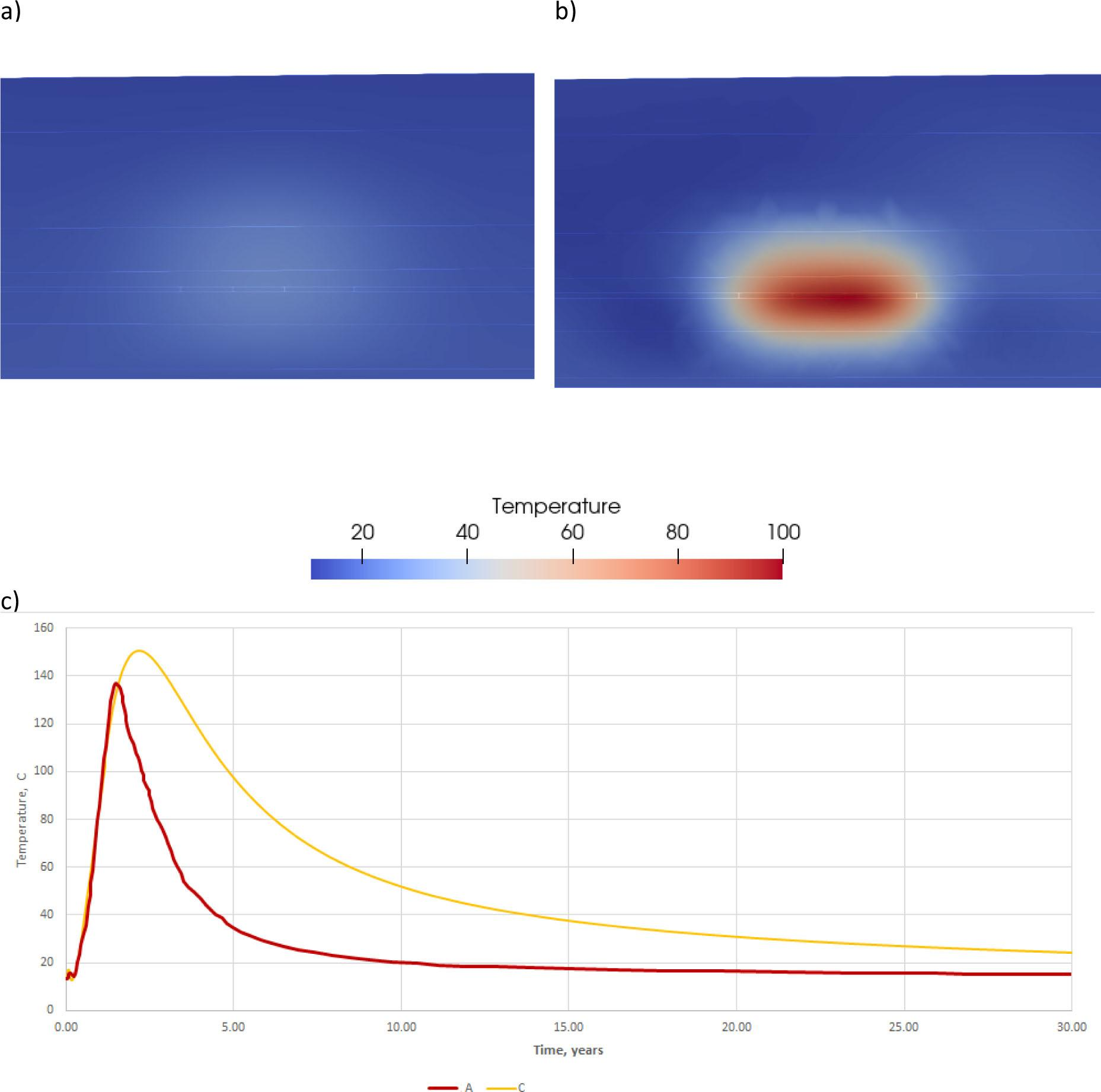A) temperature (°C) after 6 years when thermal parameters of water vapour were accepted in volume, where phase transition occurred, b) temperature in case, where no phase transition in the pores was modelled c) time course of temperature in both cases at point x = 71,5 m, y = 75,0 m, z = 63 m.

From the perspective of environmental impact, the most important issues are seepage (and migration of pollution) and subsidence. Final subsidence amounted to 5 cm in all three versions. Thanks to the application of reduced pressure with respect to the pressure in the rock mass, water flows into the generator, supplying it with water vapour and preventing propagation of pollution. The model, as presented, makes it possible to test different process parameters (such as temperature and pressure in the generator) for the purpose of determining the optimal conditions for the specific geology. Depending on the need, it is possible to use the model to analyse other issues that are not the subject of this article, such as plasticity potential in the rock mass and viscoelastic strain.

Conclusion

UCG is a problem that requires modelling of coupled fields of temperature, seepage and displacements. The proposed thermo-consolidation model enables modelling of such fields while simultaneously accounting for the presence of fluid in different states of matter within the medium's pores. Due to the different mechanical properties of both fluids – the linear stress/strain characteristic of the liquid and the non-linear characteristics of gases, there was a need for accounting gas laws in constitutive relations of the poroelastic medium.

In the proposed constitutive equations of the medium, successive terms correspond to the influence on stresses in the medium and in the fluid exerted by: strains of the medium, strains of the fluid phase, temperature changes in the solid phase and temperature changes in the fluid phase (or in the case where the medium is considered homogeneous with mean thermal parameters, the influence of the change in the medium's temperature). Terms of equations defining the influence of temperature changes on stresses use material constants of the poroelastic medium. The non-linear characteristic of gas also has an effect on heat transfer equations, in which compressibility and thermal expandability of both phases are also present.

The differences between the thermoporoelastic model (B) and proposed version with gas laws (A) are highest in vicinity of the UCG generator, so usefulness of such detailed model depends on scale – for modelling phenomena near the generator, the proposed model will be more accurate. However, time of computations is longer (about 270% compared to version B), so in modelling, only the phenomena at greater distance (such subsidence) model of thermoporoelasticity would be sufficient. In the UCG model, models for water and water vapour in the medium's pores were applied depending on the temperature and pressure. The area in which water vapour is present in pores of the medium was determined based on the Clausius–Clapeyron equation and, depending on the state of matter, constitutive equations and material parameters appropriate to gas were applied. For the assumed geology and gasification scenario, displacements as well as directions and rates of seepage were determined, which are of great importance to the safety of the process when considering migration of pollution. Under the assumed conditions, final vertical displacements of the ground's surface amounted to −5 cm, as a result of the drop in coal's strength parameters. Seepage was directed towards the generator, preventing migration of pollution in the rock mass and providing the water vapour required in the process. This model also made it possible to determine stresses in the skeleton and fluid, and to track changes of temperature-dependent parameters such as density, viscosity, heat transfer coefficient and hydraulic conductivity at selected points. This model can be modified (different geometry and material parameters can be defined in a manner that does not require high time input), and if additional data become available, it can be expanded by further material analyses.

#### Material parameter values of individual geological layers.

Layer Roof min [m] Roof max [m] f [−] N [Pa] A [Pa] α [−] ρs [Mg/m3] K [m/s] cv [J/kg/K] λ [W/m/K]
Sandstone 4.7 10.9 0.15 2.31E+09 3.46E+09 1.16E-05 2.6 5.00E-07 1100 3.1
Clayey pebble 25.2 32.7 0.28 3.90E+06 2.40E+07 6.00E-06 2.53 3.00E-06 639 2.5
Clay 50 50 0.31 1.32E+07 6.91E+07 5.80E-06 2.38 3.00E-08 566 2.1
Coal* 53 53 0.2 5.80E+08 8.70E+08 5.00E-06 1.21 5.00E-09 1250 0.5
Clay 57.2 69.8 0.4 1.28E+06 9.42E+06 5.90E-06 2.62 6.00E-08 481 2.9
Clayey sand 80.5 95 0.32 1.30E+06 9.00E+06 6.00E-06 2.49 1.20E-06 889 3.5
Clay 125.9 149.9 0.37 2.50E+06 1.85E+07 6.10E-06 2.71 7.00E-08 514 1.4
Silty clay 166.1 184 0.28 3.30E+06 1.50E+07 6.00E-06 2.66 5.00E-08 612 1.9

#### Thermal parameters of individual pore fluids and medium.

Thermal expansion coefficient 1) [1/K] Initial heat transfer coefficient [W/m/K] specific heat [J/kg/K]
Water 69·10−6 0.6 4150
Water vapour 1/T 16.2·10−3 1970
Medium - λ = (1 − f) λs + f cν = (ρ1 cνs + ρ2 cνf) / ρ

Burchart-Korol, D., Krawczyk, P., Czaplicka-Kolarz, K., Smoliński, A. (2016). Eco-efficiency of underground coal gasification (UCG) for electricity production. Fuel, 173(January), 239–246. https://doi.org/10.1016/j.fuel.2016.01.019 Burchart-KorolD. KrawczykP. Czaplicka-KolarzK. SmolińskiA. 2016 Eco-efficiency of underground coal gasification (UCG) for electricity production Fuel 173 January 239 246 https://doi.org/10.1016/j.fuel.2016.01.019 Search in Google Scholar

Meeting, A., Engineers, C., Francisco, S. (1984). Commercial project planned for underground coal gasification. Chemical and Engineering News, 62(51), 25–27. https://doi.org/10.1021/cen-v062n051.p025 MeetingA. EngineersC. FranciscoS. 1984 Commercial project planned for underground coal gasification Chemical and Engineering News 62 51 25 27 https://doi.org/10.1021/cen-v062n051.p025 Search in Google Scholar

Shafirovich, E., Varma, A. (2009). Underground coal gasification: A brief review of current status. Industrial and Engineering Chemistry Research, 48(17), 7865–7875. https://doi.org/10.1021/ie801569r ShafirovichE. VarmaA. 2009 Underground coal gasification: A brief review of current status Industrial and Engineering Chemistry Research 48 17 7865 7875 https://doi.org/10.1021/ie801569r Search in Google Scholar

Wiatowski, M., Stańczyk, K., Świadrowski, J., Kapusta, K., Cybulski, K., Krause, E., … Smoliński, A. (2012). Semi-technical underground coal gasification (UCG) using the shaft method in Experimental Mine “barbara.” Fuel, 99, 170–179. https://doi.org/10.1016/j.fuel.2012.04.017 WiatowskiM. StańczykK. ŚwiadrowskiJ. KapustaK. CybulskiK. KrauseE. SmolińskiA. 2012 Semi-technical underground coal gasification (UCG) using the shaft method in Experimental Mine “barbara.” Fuel 99 170 179 https://doi.org/10.1016/j.fuel.2012.04.017 Search in Google Scholar

Mocek, P., Pieszczek, M., Świadrowski, J., Kapusta, K., Wiatowski, M., Stańczyk, K. (2016). Pilot-scale underground coal gasification (UCG) experiment in an operating Mine “Wieczorek” in Poland. Energy, 111, 313–321. https://doi.org/10.1016/j.energy.2016.05.087 MocekP. PieszczekM. ŚwiadrowskiJ. KapustaK. WiatowskiM. StańczykK. 2016 Pilot-scale underground coal gasification (UCG) experiment in an operating Mine “Wieczorek” in Poland Energy 111 313 321 https://doi.org/10.1016/j.energy.2016.05.087 Search in Google Scholar

Friedmann, J. (2007). Fire in the Hole. Lawrence Livermore National Laboratory Science and Technology Rewiev, 4, 12–18. FriedmannJ. 2007 Fire in the Hole Lawrence Livermore National Laboratory Science and Technology Rewiev 4 12 18 Search in Google Scholar

Bhutto, A. W., Bazmi, A. A., Zahedi, G. (2013). Underground coal gasification: From fundamentals to applications. Progress in Energy and Combustion Science, 39(1), 189–214. https://doi.org/10.1016/j.pecs.2012.09.004 BhuttoA. W. BazmiA. A. ZahediG. 2013 Underground coal gasification: From fundamentals to applications Progress in Energy and Combustion Science 39 1 189 214 https://doi.org/10.1016/j.pecs.2012.09.004 Search in Google Scholar

Kostúr, K., Laciak, M., Durdan, M. (2018). Some influences of Underground Coal Gasification on the environment. Sustainability (Switzerland). https://doi.org/10.3390/su10051512 KostúrK. LaciakM. DurdanM. 2018 Some influences of Underground Coal Gasification on the environment Sustainability (Switzerland) https://doi.org/10.3390/su10051512 Search in Google Scholar

Blinderman, M. S., Blinderman, A., Taskaev, A. (2017). What makes a UCG technology ready for commercial application? Underground Coal Gasification and Combustion. Elsevier Ltd. https://doi.org/10.1016/B978-0-08-100313-8.00012-8 BlindermanM. S. BlindermanA. TaskaevA. 2017 What makes a UCG technology ready for commercial application? Underground Coal Gasification and Combustion Elsevier Ltd https://doi.org/10.1016/B978-0-08-100313-8.00012-8 Search in Google Scholar

Yang, L. (2005). Theoretical analysis of the coupling effect for the seepage field, stress field, and temperature field in underground coal gasification. Numerical Heat Transfer; Part A: Applications, 48(6), 585–606. https://doi.org/10.1080/10407780490508115 YangL. 2005 Theoretical analysis of the coupling effect for the seepage field, stress field, and temperature field in underground coal gasification Numerical Heat Transfer; Part A: Applications 48 6 585 606 https://doi.org/10.1080/10407780490508115 Search in Google Scholar

Otto, C., Kempka, T. (2015). Thermo-mechanical simulations of rock behavior in underground coal gasification show negligible impact of temperature-dependent parameters on permeability changes. Energies. https://doi.org/10.3390/en8065800 OttoC. KempkaT. 2015 Thermo-mechanical simulations of rock behavior in underground coal gasification show negligible impact of temperature-dependent parameters on permeability changes Energies https://doi.org/10.3390/en8065800 Search in Google Scholar

Li, H. zhan, Guo, G. li, Zha, J. feng, Yuan, Y. fei, Zhao, B. chen. (2016). Research on the surface movement rules and prediction method of underground coal gasification. Bulletin of Engineering Geology and the Environment. https://doi.org/10.1007/s10064-015-0809-7 LiH. zhan GuoG. li ZhaJ. feng YuanY. fei ZhaoB. chen 2016 Research on the surface movement rules and prediction method of underground coal gasification Bulletin of Engineering Geology and the Environment https://doi.org/10.1007/s10064-015-0809-7 Search in Google Scholar

Akbarzadeh Kasani, H., Chalaturnyk, R. J. (2017). Coupled reservoir and geomechanical simulation for a deep underground coal gasification project. Journal of Natural Gas Science and Engineering. https://doi.org/10.1016/j.jngse.2016.12.002 Akbarzadeh KasaniH. ChalaturnykR. J. 2017 Coupled reservoir and geomechanical simulation for a deep underground coal gasification project Journal of Natural Gas Science and Engineering https://doi.org/10.1016/j.jngse.2016.12.002 Search in Google Scholar

Pivnyak, G., Dychkovskyi, R., Bobyliov, O., Cabana, E. C., Smoliński, A. (2018). Mathematical and Geomechanical Model in Physical and Chemical Processes of Underground Coal Gasification. Solid State Phenomena, 277, 1–16. https://doi.org/10.4028/www.scientific.net/SSP.277.1 PivnyakG. DychkovskyiR. BobyliovO. CabanaE. C. SmolińskiA. 2018 Mathematical and Geomechanical Model in Physical and Chemical Processes of Underground Coal Gasification Solid State Phenomena 277 1 16 https://doi.org/10.4028/www.scientific.net/SSP.277.1 Search in Google Scholar

Uciechowska-grakowicz, A. (2018). Termokonsolidacja ośrodka porowatego z uwzględnieniem występowania fazy gazowej. Politechnika Wrocławska. Uciechowska-grakowiczA. 2018 Termokonsolidacja ośrodka porowatego z uwzględnieniem występowania fazy gazowej Politechnika Wrocławska Search in Google Scholar

Strzelecki, T., Bartlewska-Urban, M., Kaźmierczak, A., Overchenko, L., Strzelecki, M., Uciechowska-Grakowicz, A. (2018). Mechanika ośrodków porowatych. Wrocław: Dolnośląskie Wydawnictwo Edukacyjne. StrzeleckiT. Bartlewska-UrbanM. KaźmierczakA. OverchenkoL. StrzeleckiM. Uciechowska-GrakowiczA. 2018 Mechanika ośrodków porowatych Wrocław Dolnośląskie Wydawnictwo Edukacyjne Search in Google Scholar

Biot, M. A. (1941). Reprinted Series General Theory of Three-Dimensional Consolidation. Journal of Applied Physics, 12(2), 155–164. https://doi.org/10.1063/1.1712886 BiotM. A. 1941 Reprinted Series General Theory of Three-Dimensional Consolidation Journal of Applied Physics 12 2 155 164 https://doi.org/10.1063/1.1712886 Search in Google Scholar

Biot, M. A. (1955). Theory of elasticity and consolidation for a porous anisotropic solid. Journal of Applied Physics, 26(2), 182–185. https://doi.org/10.1063/1.1721956 BiotM. A. 1955 Theory of elasticity and consolidation for a porous anisotropic solid Journal of Applied Physics 26 2 182 185 https://doi.org/10.1063/1.1721956 Search in Google Scholar

Ulm, F.-J., Constantinides, G., Heukamp, F. H. (2004). Is concrete a poromechanics materials?—A multiscale investigation of poroelastic properties. Materials and Structures. https://doi.org/10.1007/BF02481626 UlmF.-J. ConstantinidesG. HeukampF. H. 2004 Is concrete a poromechanics materials?—A multiscale investigation of poroelastic properties Materials and Structures https://doi.org/10.1007/BF02481626 Search in Google Scholar

Moeendarbary, E., Valon, L., Fritzsche, M., Harris, A. R., Moulding, D. A., Thrasher, A. J., … Charras, G. T. (2013). The cytoplasm of living cells behaves as a poroelastic material. Nature Materials. https://doi.org/10.1038/nmat3517 MoeendarbaryE. ValonL. FritzscheM. HarrisA. R. MouldingD. A. ThrasherA. J. CharrasG. T. 2013 The cytoplasm of living cells behaves as a poroelastic material Nature Materials https://doi.org/10.1038/nmat3517 Search in Google Scholar

Berger, L., Bordas, R., Burrowes, K., Grau, V., Tavener, S., Kay, D. (2016). A poroelastic model coupled to a fluid network with applications in lung modelling. International Journal for Numerical Methods in Biomedical Engineering. https://doi.org/10.1002/cnm.2731 BergerL. BordasR. BurrowesK. GrauV. TavenerS. KayD. 2016 A poroelastic model coupled to a fluid network with applications in lung modelling International Journal for Numerical Methods in Biomedical Engineering https://doi.org/10.1002/cnm.2731 Search in Google Scholar

Coussy, O. (2007). Revisiting the constitutive equations of unsaturated porous solids using a Lagrangian saturation concept. International Journal for Numerical and Analytical Methods in Geomechanics. https://doi.org/10.1002/nag.613 CoussyO. 2007 Revisiting the constitutive equations of unsaturated porous solids using a Lagrangian saturation concept International Journal for Numerical and Analytical Methods in Geomechanics https://doi.org/10.1002/nag.613 Search in Google Scholar

Coussy, O. (2010). Mechanics and Physics of Porous Solids. Mechanics and Physics of Porous Solids. https://doi.org/10.1002/9780470710388 CoussyO. 2010 Mechanics and Physics of Porous Solids Mechanics and Physics of Porous Solids https://doi.org/10.1002/9780470710388 Search in Google Scholar

Derski, W. (1975). Zarys Mechaniki Ośrodków Ciągłych. PWN Warszawa. DerskiW. 1975 Zarys Mechaniki Ośrodków Ciągłych PWN Warszawa Search in Google Scholar

Strzelecki, T., Kostecki, S., Żak, S. (2008). Modelowanie przepływów przez ośrodki porowate. Wrocław: DWE. StrzeleckiT. KosteckiS. ŻakS. 2008 Modelowanie przepływów przez ośrodki porowate Wrocław DWE Search in Google Scholar

Coussy, O. (2004). Poromechanics. John Wiley & Sons, Ltd. CoussyO. 2004 Poromechanics John Wiley & Sons, Ltd Search in Google Scholar

Gawin, D., Baggio, P., Schrefler, B. A. (1995). Coupled heat, water and gas flow in deformable porous media. International Journal for Numerical Methods in Fluids, 20(8–9), 969–987. https://doi.org/10.1002/fld.1650200817 GawinD. BaggioP. SchreflerB. A. 1995 Coupled heat, water and gas flow in deformable porous media International Journal for Numerical Methods in Fluids 20 8–9 969 987 https://doi.org/10.1002/fld.1650200817 Search in Google Scholar

Bartlewska-Urban, M., Strzelecki, T. (2014). Numerical Calculation of Deformation of Three Dimensional Sample in Triaxial Apparatus Under External Load and Temperature Field. Studia Geotechnica et Mechanica, 35(1), 27–39. https://doi.org/10.2478/sgem-2013-0003 Bartlewska-UrbanM. StrzeleckiT. 2014 Numerical Calculation of Deformation of Three Dimensional Sample in Triaxial Apparatus Under External Load and Temperature Field Studia Geotechnica et Mechanica 35 1 27 39 https://doi.org/10.2478/sgem-2013-0003 Search in Google Scholar

Strzelecki, M. (2016). Model termo-filtracji w obszarze oddziaływania generatora zgazowania węgla. StrzeleckiM. 2016 Model termo-filtracji w obszarze oddziaływania generatora zgazowania węgla Search in Google Scholar

Liu, J., Liang, X., Xue, Y., Yao, K., Fu, Y. (2020). Numerical evaluation on multiphase flow and heat transfer during thermal stimulation enhanced shale gas recovery. Applied Thermal Engineering, 178, 115554. https://doi.org/10.1016/j.applthermaleng.2020.115554 LiuJ. LiangX. XueY. YaoK. FuY. 2020 Numerical evaluation on multiphase flow and heat transfer during thermal stimulation enhanced shale gas recovery Applied Thermal Engineering 178 115554 https://doi.org/10.1016/j.applthermaleng.2020.115554 Search in Google Scholar

Nowacki, W. (1975). Teoria sprężystości. PWN Warszawa. NowackiW. 1975 Teoria sprężystości PWN Warszawa Search in Google Scholar

Suárez-arriaga, M. C. (2010). Thermo-poroelasticity in geothermics, formulated in four dimensions La termoporoelasticidad en geotermia, definida en cuatro dimensiones, 23(2), 41–50. Suárez-arriagaM. C. 2010 Thermo-poroelasticity in geothermics, formulated in four dimensions La termoporoelasticidad en geotermia, definida en cuatro dimensiones 23 2 41 50 Search in Google Scholar

Tran, D., Settari, A., Nghiem, L. (2004). New Iterative Coupling Between a Reservoir Simulator and a Geomechanics Module. SPE Journal. https://doi.org/10.2118/88989-PA TranD. SettariA. NghiemL. 2004 New Iterative Coupling Between a Reservoir Simulator and a Geomechanics Module SPE Journal https://doi.org/10.2118/88989-PA Search in Google Scholar

Bary, B., De Morais, M. V. G., Poyet, S., Durand, S. (2012). Simulations of the thermo-hydro-mechanical behaviour of an annular reinforced concrete structure heated up to 200°C. Engineering Structures, 36, 302–315. https://doi.org/10.1016/j.engstruct.2011.12.007 BaryB. De MoraisM. V. G. PoyetS. DurandS. 2012 Simulations of the thermo-hydro-mechanical behaviour of an annular reinforced concrete structure heated up to 200°C Engineering Structures 36 302 315 https://doi.org/10.1016/j.engstruct.2011.12.007 Search in Google Scholar

Bartlewska-Urban, M., Zombroń, M., Strzelecki, T. (2016). Numerical analysis of road pavement thermal deformability, based on biot viscoelastic model of porous medium. Studia Geotechnica et Mechanica, 38(1), 15–22. https://doi.org/10.1515/sgem-2016-0002 Bartlewska-UrbanM. ZombrońM. StrzeleckiT. 2016 Numerical analysis of road pavement thermal deformability, based on biot viscoelastic model of porous medium Studia Geotechnica et Mechanica 38 1 15 22 https://doi.org/10.1515/sgem-2016-0002 Search in Google Scholar

Lecampion, B. (2013). A macroscopic poromechanical model of cement hydration. European Journal of Environmental and Civil Engineering. https://doi.org/10.1080/19648189.2013.768554 LecampionB. 2013 A macroscopic poromechanical model of cement hydration European Journal of Environmental and Civil Engineering https://doi.org/10.1080/19648189.2013.768554 Search in Google Scholar

Néron, D., Dureisseix, D. (2008). A computational strategy for thermo-poroelastic structures with a time-space interface coupling. International Journal for Numerical Methods in Engineering. https://doi.org/10.1002/nme.2283 NéronD. DureisseixD. 2008 A computational strategy for thermo-poroelastic structures with a time-space interface coupling International Journal for Numerical Methods in Engineering https://doi.org/10.1002/nme.2283 Search in Google Scholar

Rosen, M. A., Reddy, B. V., Self, S. J. (2017). Underground coal gasification (UCG) modeling and analysis. Underground Coal Gasification and Combustion. Elsevier Ltd. https://doi.org/10.1016/B978-0-08-100313-8.00011-6 RosenM. A. ReddyB. V. SelfS. J. 2017 Underground coal gasification (UCG) modeling and analysis Underground Coal Gasification and Combustion Elsevier Ltd https://doi.org/10.1016/B978-0-08-100313-8.00011-6 Search in Google Scholar

Biot, M. A. (1956). Theory of Propagation of Elastic Waves in a Fluid-Saturated Porous Solid. I. Low Frequency Range. The Journal of the Acoustical Society of America. https://doi.org/10.1063/1.1721956 BiotM. A. 1956 Theory of Propagation of Elastic Waves in a Fluid-Saturated Porous Solid. I. Low Frequency Range The Journal of the Acoustical Society of America https://doi.org/10.1063/1.1721956 Search in Google Scholar

Xue, Y., Teng, T., Dang, F., Ma, Z., Wang, S., Xue, H. (2020). Productivity analysis of fractured wells in reservoir of hydrogen and carbon based on dual-porosity medium model. International Journal of Hydrogen Energy, 45(39), 20240–20249. https://doi.org/10.1016/j.ijhydene.2019.11.146 XueY. TengT. DangF. MaZ. WangS. XueH. 2020 Productivity analysis of fractured wells in reservoir of hydrogen and carbon based on dual-porosity medium model International Journal of Hydrogen Energy 45 39 20240 20249 https://doi.org/10.1016/j.ijhydene.2019.11.146 Search in Google Scholar

Uciechowska-Grakowicz, A., Strzelecki, T. (2017). Non-Isothermal Constitutive Relations and Heat Transfer Equations of a Two-Phase Medium. Studia Geotechnica et Mechanica, 39(3), 67–78. https://doi.org/10.1515/sgem-2017-0031 Uciechowska-GrakowiczA. StrzeleckiT. 2017 Non-Isothermal Constitutive Relations and Heat Transfer Equations of a Two-Phase Medium Studia Geotechnica et Mechanica 39 3 67 78 https://doi.org/10.1515/sgem-2017-0031 Search in Google Scholar

Uciechowska-Grakowicz, A., Strzelecki, T. (2016). Numerical model of heat transfer in three phases of the poroelastic medium. Studia Geotechnica et Mechanica, 38(2), 53–59. https://doi.org/10.1515/sgem-2016-0019 Uciechowska-GrakowiczA. StrzeleckiT. 2016 Numerical model of heat transfer in three phases of the poroelastic medium Studia Geotechnica et Mechanica 38 2 53 59 https://doi.org/10.1515/sgem-2016-0019 Search in Google Scholar

Strzelecki, T. (2006). Równania termokonsolidacji gruntów i skał: Geotechnika i budownictwo specjalne. In XXIX Zimowa Szkoła Mechaniki Górotworu i Geoinzynierii, Kraków Krynica 12–17 marca 2006 (pp. 285–299). wyd. Katedry Geomechaniki, Budownictwa i Geotechniki AGH. StrzeleckiT. 2006 Równania termokonsolidacji gruntów i skał: Geotechnika i budownictwo specjalne In XXIX Zimowa Szkoła Mechaniki Górotworu i Geoinzynierii Kraków Krynica 12–17 marca 2006 285 299 wyd. Katedry Geomechaniki, Budownictwa i Geotechniki AGH Search in Google Scholar

Biot, M. A., Willis, D. G. (1957). The Elastic Coefficients of the Theory of Consolidation. Journal of Applied Mechanics. https://doi.org/10.1002/9780470172766.ch13 BiotM. A. WillisD. G. 1957 The Elastic Coefficients of the Theory of Consolidation Journal of Applied Mechanics https://doi.org/10.1002/9780470172766.ch13 Search in Google Scholar

Blachowski, J. (2015). Methodology for assessment of the accessibility of a brown coal deposit with Analytical Hierarchy Process and Weighted Linear Combination. Environmental Earth Sciences, 74(5), 4119–4131. https://doi.org/10.1007/s12665-015-4461-0 BlachowskiJ. 2015 Methodology for assessment of the accessibility of a brown coal deposit with Analytical Hierarchy Process and Weighted Linear Combination Environmental Earth Sciences 74 5 4119 4131 https://doi.org/10.1007/s12665-015-4461-0 Search in Google Scholar

Nowak, J., Kudelko, J. (2013). LGOM region as a perspective power energy basin and implementation of innovative lignite development methods. Mineral Economics, 25(2–3), 65–70. https://doi.org/10.1007/s13563-012-0024-y NowakJ. KudelkoJ. 2013 LGOM region as a perspective power energy basin and implementation of innovative lignite development methods Mineral Economics 25 2–3 65 70 https://doi.org/10.1007/s13563-012-0024-y Search in Google Scholar

Blinderman, M. S., Jones, R. M. (2002). The Chinchilla IGCC Project to Date: Underground Coal Gasification and Environment. Gasification Technologies Conference, San Francisco, USA, October 27–30, 14. Retrieved from http://www.lincenergy.com/data/info_sheets/u3-fact.pdf BlindermanM. S. JonesR. M. 2002 The Chinchilla IGCC Project to Date: Underground Coal Gasification and Environment Gasification Technologies Conference San Francisco, USA October 27–30 14 Retrieved from http://www.lincenergy.com/data/info_sheets/u3-fact.pdf Search in Google Scholar

Yang, L. H. (2008). Model test on Underground Coal Gasification (UCG) with low-pressure fire Seepage push-through. Part I: Test conditions and air fire seepage. Energy Sources, Part A: Recovery, Utilization and Environmental Effects, 30(17), 1587–1594. https://doi.org/10.1080/15567030802112102 YangL. H. 2008 Model test on Underground Coal Gasification (UCG) with low-pressure fire Seepage push-through. Part I: Test conditions and air fire seepage Energy Sources, Part A: Recovery, Utilization and Environmental Effects 30 17 1587 1594 https://doi.org/10.1080/15567030802112102 Search in Google Scholar

• #### Usefulness of the CPTU method in evaluating shear modulus G0 changes in the subsoil

Recommended articles from Trend MD